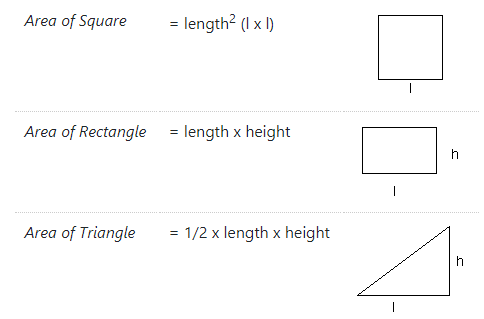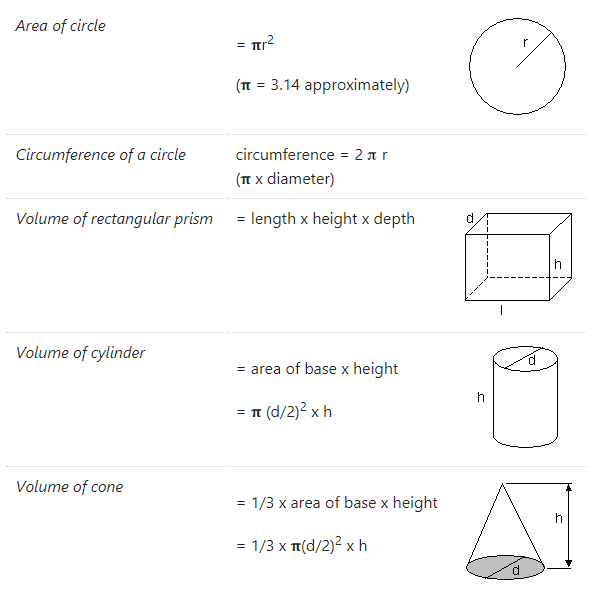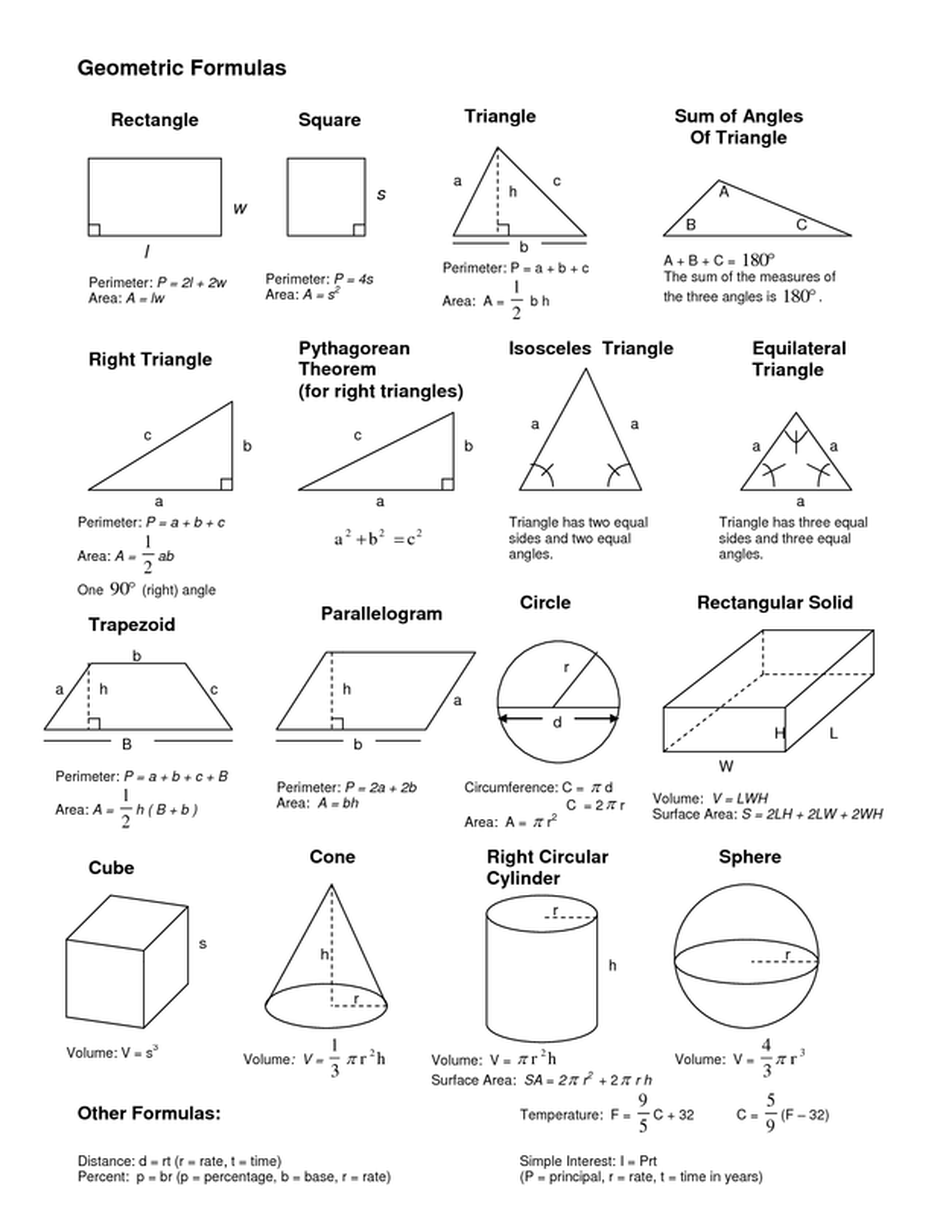# Geometry Formulas for Class 12, 11, 10, 9, 8

Geometry is a subdivision of Mathematics and is all about size, shapes, relative position of figures. It was predominant in earlier times and is a practical way to find out lengths, Volumes, and Areas. It is split into two parts namely Plane Geometry and Solid Geometry. There are many Geometrical Formulas related to height, width, radius, areas, and Volumes.

We tried mentioning some of the Geometry Formulas for Classes 8 to 12 that can be used to solve problems. If you get stumped while solving a problem this is the place you should look into. Some geometric formulas are rather complicated and you might have hardly heard of them. In addition, we also provided basic maths formulas for Classes 12, 11, 10, 9, 8 which are used in our day to day lives to calculate the space, length and so on.

Main Concern of every student about the subject is to learn about Geometry Formulas. There are some basic formulas that you really need to memorize and you are expected to learn them. To make it easy for you we have sorted several Geometry Formulas under the parent topics and you can use them during your preparation.

Students of Class 12, 11, 10, 9, 8 can make their preparation effective with the handy formula list prevailing. Use them while solving questions and come to a conclusion easily with a simple approach. Download the Geometry Formulas for Class 8 to Class 12 PDF for free and aid your preparation. Refer to the further modules to avail the quick links to Geometry Formulas PDF and solve the questions and answers in Geometry easily.

Here is a list of several most important geometry formulas that you use for solving various problems.

## Basic Geometry Formulas

• Perimeter of a Square = P = 4a

Where a = Length of the sides of a Square

• Perimeter of a Rectangle = P = 2(l+b)

Where, l = Length ; b = Breadth

• Area of a Square = A = a2

Where a = Length of the sides of a Square

• Area of a Rectangle = A = l×b

Where, l = Length ; b = Breadth

• Area of a Triangle = A = ½×b×h

Where, b = base of the triangle ; h = height of the triangle• Area of a Trapezoid = A = ½×(b1 + b2)×h

Where, b1 & b2 are the bases of the Trapezoid ; h = height of the Trapezoid

• Area of a Circle = A = π×r2
• Circumference of a Circle = A = 2πr

Where, r = Radius of the Circle

• Surface Area of a Cube = S = 6a2

Where, a = Length of the sides of a Cube• Curved surface area of a Cylinder  = 2πrh
• Total surface area of a Cylinder = 2πr(r + h)
• Volume of a Cylinder = V = πr2h

Where, r = Radius of the base of the Cylinder ; h = Height of the Cylinder

• Curved surface area of a cone =  πrl
• Total surface area of a cone = πr(r+l) = πr[r+√(h2+r2)]
• Volume of a Cone = V = ⅓×πr2h

Where, r = Radius of the base of the Cone, h = Height of the Cone

• Surface Area of a Sphere = S = 4πr2
• Volume of a Sphere = V = 4/3×πr3

Where, r = Radius of the Sphere### Geometric Formulas

Get Common Geometry Formulas for Class 8 to 12 for various shapes and figures. Students can Download Geometric Formulas Cheat Sheet PDF for free of cost.### FAQs on Geometry Formulas

1. Where do you get all formula of Geometry?

You can get the Geometry Formula for Classes 12, 11, 10, 9, 8 from our page. Access the quick links available here in PDF format and know the formulas for all the topics.

2. Can you give some important formulas on Geometry?

Students of Class 8 to Class 12 will find information related to basic and important formulas of geometry that will help you score better grades in the exam from here.